• 最大后验估计(maximum a posteriori estimation) 上面的最大似然估计MLE其实就是求一组能够使似然函数最大的参数，即 θ^ML(x)=arg⁡max⁡θf(x∣θ) ⁣\displaystyle {\hat {\theta }}_{\mathrm {ML} }(x)=\arg \...
项目github地址：bitcarmanlee easy-algorithm-interview-and-practice
欢迎大家star，留言，一起学习进步
1.什么是参数
在机器学习中，我们经常使用一个模型来描述生成观察数据的过程。例如，我们可以使用一个随机森林模型来分类客户是否会取消订阅服务（称为流失建模），或者我们可以用线性模型根据公司的广告支出来预测公司的收入（这是一个线性回归的例子）。每个模型都包含自己的一组参数，这些参数最终定义了模型本身。
我们可以把线性模型写成 y = mx + c 的形式。在广告预测收入的例子中，x 可以表示广告支出，y 是产生的收入。m 和 c 则是这个模型的参数。这些参数的不同值将在坐标平面上给出不同的直线（见下图）。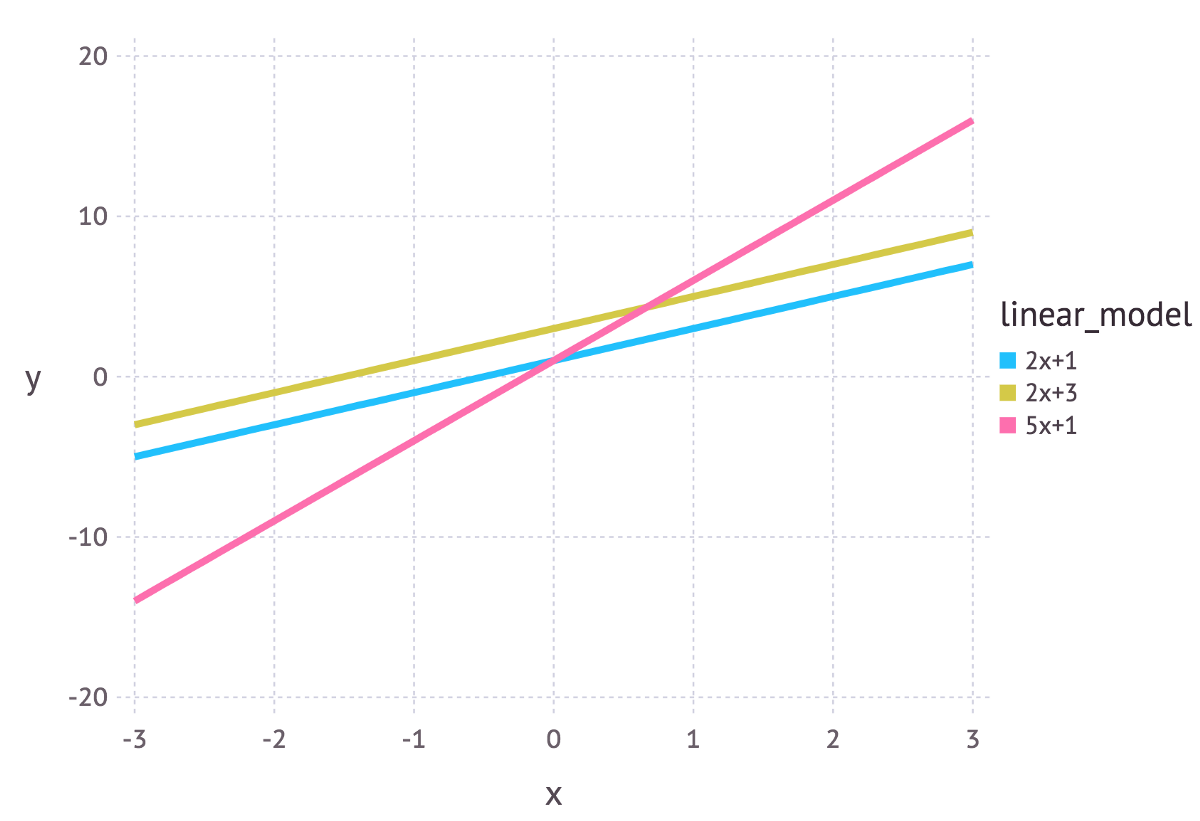2.参数估计的方法
就是根据样本统计量的数值对总体参数进行估计的过程。根据参数估计的性质不同，可以分成两种类型：点估计和区间估计。
点估计就是用样本统计量的某一具体数值直接推断未知的总体参数。例如，在进行有关小学生身高的研究中，随机抽取1000名小学生并计算出他们的平均身高为1.45米。如果直接用这个1.45米代表所有小学生的平均身高，那么这种估计方法就是点估计。
而对总体参数进行点估计常用的方法有两种：矩估计与最大似然估计，其中最大似然估计就是我们实际中使用非常广泛的一种方法。
按这两种方法对总体参数进行点估计，能够得到相对准确的结果。如用样本均值X估计总体均值，或者用样本标准差S估计总体标准差σ。
但是，点估计有一个不足之处，即这种估计方法不能提供估计参数的估计误差大小。对于一个总体来说，它的总体参数是一个常数值，而它的样本统计量却是随机变量。当用随机变量去估计常数值时，误差是不可避免的，只用一个样本数值去估计总体参数是要冒很大风险的。因为这种误差风险的存在，并且风险的大小还未知，所以，点估计主要为许多定性研究提供一定的参考数据，或在对总体参数要求不精确时使用，而在需要用精确总体参数的数据进行决策时则很少使用。
区间估计就是在推断总体参数时，还要根据统计量的抽样分布特征，估计出总体参数的一个区间，而不是一个数值，并同时给出总体参数落在这一区间的可能性大小，概率的保证。还是举小学生身高的例子，如果用区间估计的方法推断小学生身高，则会给出以下的表达：根据样本数据，估计小学生的平均身高在1.4~1.5米之间，置信程度为95%，这种估计就属于区间估计。
3.概率与统计的区别
概率（probabilty）和统计（statistics）看似两个相近的概念，其实研究的问题刚好相反。
概率研究的问题是，已知一个模型和参数，怎么去预测这个模型产生的结果的特性（例如均值，方差，协方差等等）。 举个例子，我想研究怎么养猪（模型是猪），我选好了想养的品种、喂养方式、猪棚的设计等等（选择参数），我想知道我养出来的猪大概能有多肥，肉质怎么样（预测结果）。
统计研究的问题则相反。统计是，有一堆数据，要利用这堆数据去预测模型和参数。仍以猪为例。现在我买到了一堆肉，通过观察和判断，我确定这是猪肉（这就确定了模型。在实际研究中，也是通过观察数据推测模型是／像高斯分布的、指数分布的、拉普拉斯分布的等等），然后，可以进一步研究，判定这猪的品种、这是圈养猪还是跑山猪还是网易猪，等等（推测模型参数）。
一句话总结：概率是已知模型和参数，推数据。统计是已知数据，推模型和参数。
显然，对于最大似然估计，最大后验估计，贝叶斯估计来说，都属于统计的范畴。
4.最大似然估计(maximum likelihood estimates，MLE)
前文提到，最大似然估计(maximum likelihood estimates，MLE)是实际中使用非常广泛的一种方法，用我们老师的一句最简单的话来总结最大似然估计，就是“谁大像谁”。
说到最大似然估计与最大后验估计，最好的例子自然就是抛硬币了。本文也不免俗，同样以抛硬币作为例子。
于是我们拿这枚硬币抛了10次，得到的数据X是：反正正正正反正正正反。我们想求的正面概率θ是模型参数，而抛硬币模型我们可以假设是二项分布。
在概率论和统计学中，二项分布（Binomial distribution）是n个独立的是/非试验中成功的次数的离散概率分布，其中每次试验的成功概率为p。这样的单次成功/失败试验又称为伯努利试验。实际上，当n = 1时，二项分布就是伯努利分布。
伯努利分布（Bernoulli distribution，又名两点分布或者0-1分布，是一个离散型概率分布，为纪念瑞士科学家雅各布·伯努利而命名。)若伯努利试验成功，则伯努利随机变量取值为0。记其成功概率为 $p(0{\leq }p{\leq }1)$,失败概率为 $q=1-p$。
对于伯努利分布来说：
概率质量函数为：
KaTeX parse error: Undefined control sequence: \mbox at position 66: …egin{matrix}p&{\̲m̲b̲o̲x̲{if }}x=1,\\q\ …
期望为：
$\displaystyle \operatorname {E} [X]=\sum _{i=0}^{1}x_{i}f_{X}(x)=0+p=p$
方差为:
$\displaystyle \operatorname {var} [X]=\sum _{i=0}^{1}(x_{i}-E[X])^{2}f_{X}(x)=(0-p)^{2}(1-p)+(1-p)^{2}p=p(1-p)=pq$
而如果X ~ B(n, p)（也就是说，X是服从二项分布的随机变量）
一般的二项分布是n次独立的伯努利试验的和。它的期望值和方差分别等于每次单独试验的期望值和方差的和：
$\displaystyle \mu _{n}=\sum _{k=1}^{n}\mu =np,\qquad \sigma _{n}^{2}=\sum _{k=1}^{n}\sigma ^{2}=np(1-p).$
回到抛硬币的例子，出现实验结果X的似然函数是什么呢？
$f(X, \theta) = \theta^7(1-\theta)^3$
需要注意的是，上面只是个关于$\theta$的函数。而最大似然估计，很明显是要最大化这个函数。可以看一下这个函数的图像：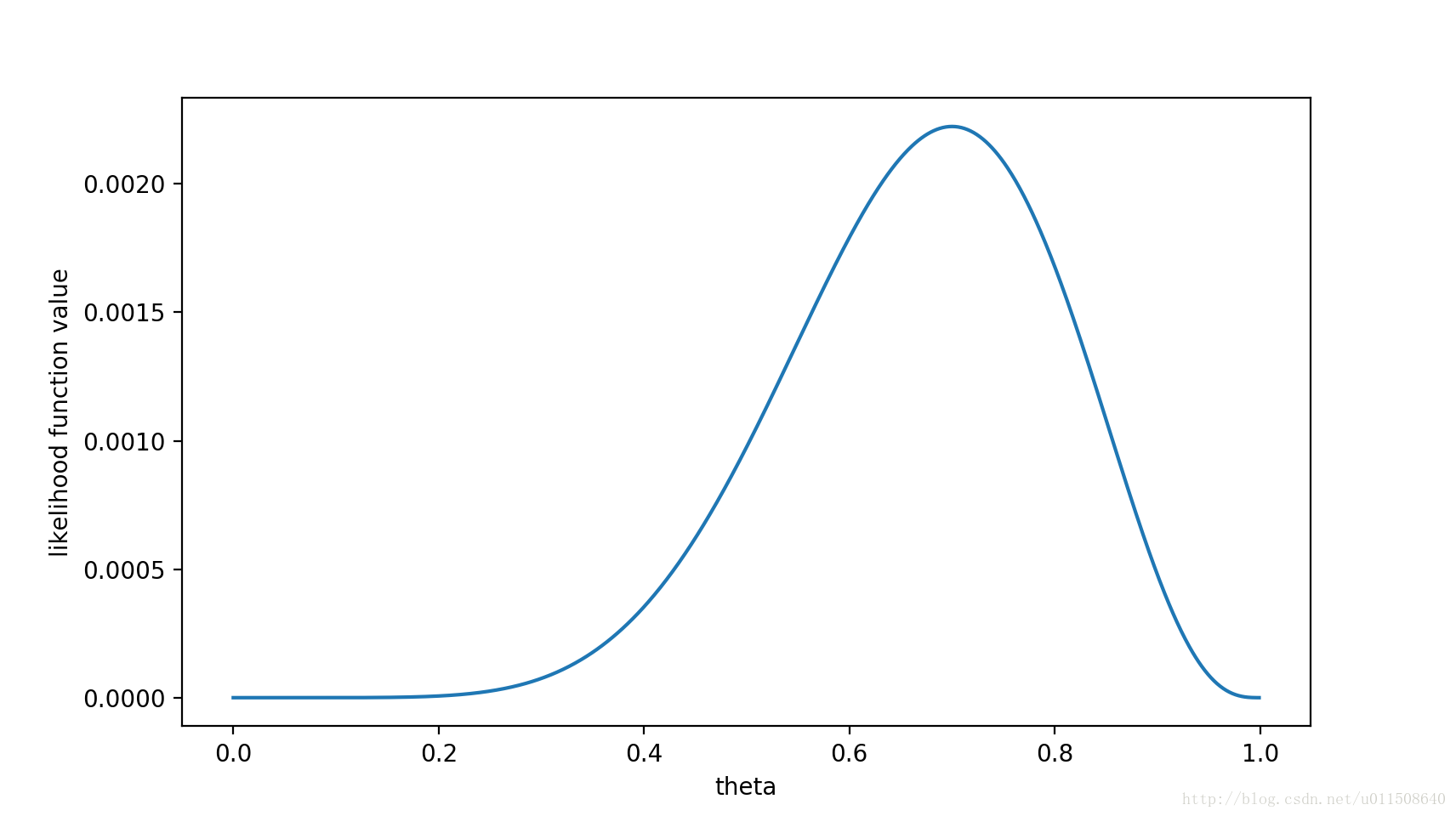容易得出，在$\theta=0.7$时，似然函数能取到最大值。
当然实际中我们一般不会画图，而是通过更为简洁的数学手段来处理。
首先我们取对数似然函数，这样更方便后续的数学运算：
$ln(f(X, \theta)) = ln(\theta^7(1-\theta)^3) = 7ln(\theta) + 3ln(1-\theta)$
对对数似然函数求导：
$ln'(f(X, \theta)) = \frac{7}{\theta} - \frac{3}{1-\theta}$
令导数为0:
$7(1-\theta) - 3\theta = 0$
最终求得：
$\theta = 0.7$
这样，我们已经完成了对
的最大似然估计。即，抛10次硬币，发现7次硬币正面向上，最大似然估计认为正面向上的概率是0.7。是不是非常直接，非常简单粗暴？没错，就是这样，谁大像谁！
说到这里为止，可能很多同学不以为然：你这不坑爹嘛？只要硬币一枚正常硬币，不存在作弊情况，正面朝上的概率必然为0.5么，你这怎么就忽悠我们是0.7呢。OK，如果你这么想，恭喜你，那你就天然包含了贝叶斯学派的思想！我们所谓的正常硬币向上的概率为0.5，就是贝叶斯里的先验概率。
5.最大后验估计(maximum a posteriori estimation)
上面的最大似然估计MLE其实就是求一组能够使似然函数最大的参数，即
$\displaystyle {\hat {\theta }}_{\mathrm {ML} }(x)=\arg \max _{\theta }f(x|\theta )\!$
如果我们把问题稍微弄复杂一点，如果这个参数$\theta$有一个先验概率呢？比如上面的例子中，实际生活经验告诉我们，硬币一般都是均匀的，也就是$\theta=0.5$的概率最大，那么这个参数该怎么估计？
这个时候就用到了我们的最大后验概率MAP。MAP的基础是贝叶斯公式：
$p(\theta|x) = \frac{p(x|\theta)\times p(\theta)}{P(x)}$
其中，$p(x|\theta)$就是之前讲的似然函数，$p(\theta)$是先验概率，是指在没有任何实验数据的时候对参数 $\theta$的经验判断，对于一个硬币，大概率认为他是正常的，正面的概率为0.5的可能性最大。
MAP优化的就是一个后验概率，即给定了观测值以后使后验概率最大：
KaTeX parse error: No such environment: align* at position 7: \begin{̲a̲l̲i̲g̲n̲*̲}̲
{\hat {\theta …
从上面公式可以看出，$p(x|\theta)$是似然函数，而$p(\theta)$就是先验概率。对其取对数：
KaTeX parse error: No such environment: align* at position 7: \begin{̲a̲l̲i̲g̲n̲*̲}̲
\arg \max _{\t…
通过MAP最终的式子不难看出，MAP就是多个作为因子的先验概率$P(\theta)$。这个$p(\theta)$可以是任何的概率分布，比如高斯分布，比如也可以是$\beta$分布。比如$\beta(5,2)$的概率分布图如下：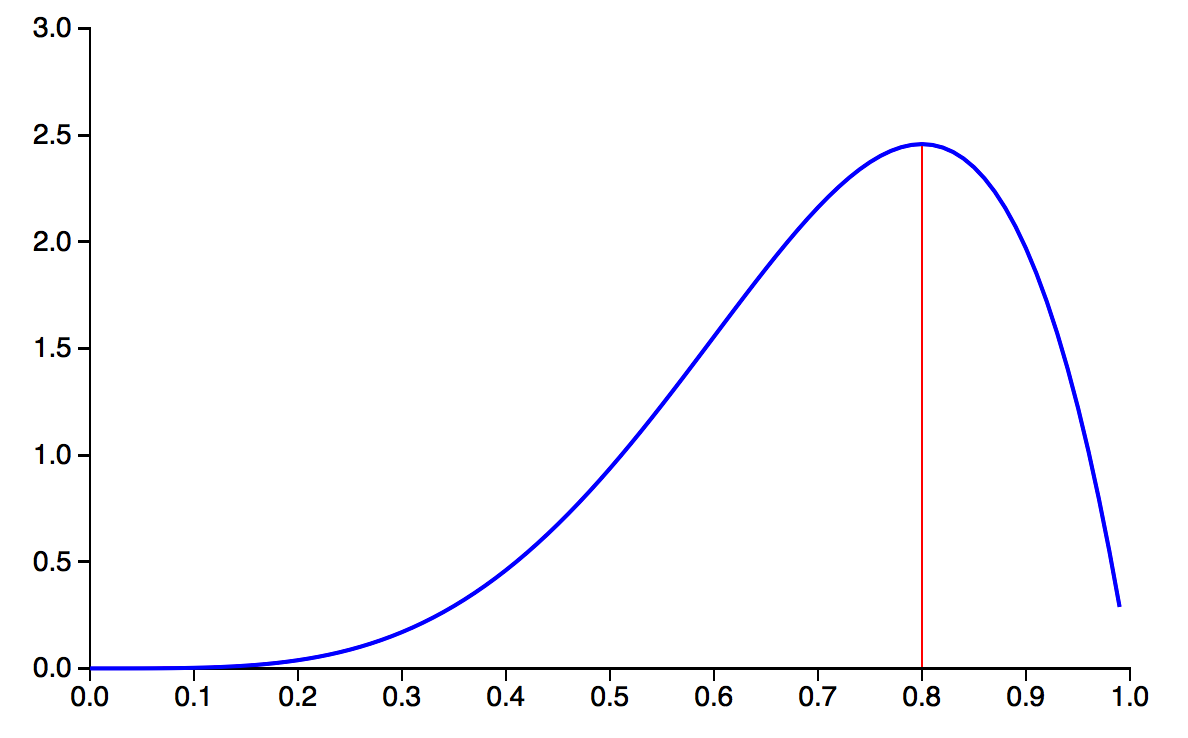如果将这个概率分布作为$p(\theta)$，那么我们在还未抛硬币前，便认为$\theta$很可能接近于0.8，而不大可能是个很小的值或是一个很大的值。换言之，我们在抛硬币前，便估计这枚硬币更可能有0.8的概率抛出正面。
那么问题就来了，为什么我们要用$\beta$分布来描述先验概率呢？
首先一点，通过调节 Beta 分布中的a和b,你可以让这个概率分布变成各种你想要的形状！Beta 分布已经很足够表达我们事先对$\theta$的估计了。
更重要的一点是，如果使用Beta 分布，会让之后的计算更加方便。因为有如下结论：
$p(\theta)$是个Beta分布，那么在观测到"X = 抛10次硬币出现7次正面"的事件后，$p(\theta|X)$仍然是个Beta分布，只不过此时概率分布的形状因为有了观测事件而发生了变化！此时有
$p(\theta|X) = Beta(\theta|a+3, b+2)$
换句话说，数据观测前后，对$\theta$的估计的概率分布均为 Beta 分布，这就是为什么使用 Beta 分布方便我们计算的原因。当我们得知$p(\theta|X) = Beta(\theta|a+3, b+2)$后，只要根据 Beta 分布的特性，得出$\theta$最有可能等于多少了。即$\theta$等于多少时，观测后得到的 Beta 分布有最大的概率密度）。
到此为止，我们可以得到“共轭性”的真正含义了！后验概率分布（正⽐于先验和似然函数的乘积）拥有与先验分布相同的函数形式。这个性质被叫做共轭性（Conjugacy）。共轭先验（conjugate prior）有着很重要的作⽤。它使得后验概率分布的函数形式与先验概率相同，因此使得贝叶斯分析得到了极⼤的简化。例如，二项分布的参数之共轭先验就是我们前面介绍的 Beta 分布。多项式分布的参数之共轭先验则是 Dirichlet 分布，⽽⾼斯分布的均值之共轭先验是另⼀个⾼斯分布。
总的来说，对于给定的概率分布$p(X|\theta)$，我们可以寻求一个与该似然函数$p(X|\theta)$共轭的先验分布$p(\theta)$，如此一来后验分布$p(\theta|X)$就会同先验分布具有相同的函数形式。而且对于任何指数族成员来说，都存在有一个共轭先验。
6.贝叶斯估计
贝叶斯估计是在MAP上做进一步拓展，此时不直接估计参数的值，而是允许参数服从一定概率分布。回忆下贝叶斯公式：
$p(\theta|x) = \frac{p(x|\theta)\times p(\theta)}{P(x)}$
现在我们不要求后验概率最大，这个时候就需要求$p(X)$，即观察到的X的概率。一般来说，用全概率公式可以求$p(X)$
$p(X) = \int p(X | \theta)p(\theta)d\theta$
那么如何用贝叶斯估计来预测呢？如果我们想求一个值 x’ 的概率，可以用下面的方法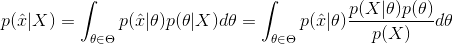7.什么时候 MAP 估计与最大似然估计相等？
当先验分布均匀之时，MAP 估计与 MLE 相等。直观讲，它表征了最有可能值的任何先验知识的匮乏。在这一情况中，所有权重分配到似然函数，因此当我们把先验与似然相乘，由此得到的后验极其类似于似然。因此，最大似然方法可被看作一种特殊的 MAP。
如果先验认为这个硬币是概率是均匀分布的，被称为无信息先验( non-informative prior )，通俗的说就是“让数据自己说话”，此时贝叶斯方法等同于频率方法。
随着数据的增加，先验的作用越来越弱，数据的作用越来越强，参数的分布会向着最大似然估计靠拢。而且可以证明，最大后验估计的结果是先验和最大似然估计的凸组合。
参考文献：
1.https://blog.csdn.net/baimafujinji/article/details/51374202
2.https://blog.csdn.net/yt71656/article/details/42585873
3.https://www.jiqizhixin.com/articles/2018-01-09-6
4.https://zh.wikipedia.org/zh-hans/二項分佈
5.https://zh.wikipedia.org/wiki/伯努利分布
6.Pattern Recognition And Machine Learning


展开全文最大似然估计 贝叶斯估计 参数估计
• 最大似然估计、贝叶斯估计和最大后验估计参数估计最大似然估计概述前提假设核心思想推导过程求解过程最大后验估计概述前提假设核心思想推导过程贝叶斯估计最大似然估计和最大后验估计的对比 参数估计 参数估计是根据...
最大似然估计、贝叶斯估计和最大后验估计参数估计最大似然估计概述前提假设核心思想推导过程求解过程最大后验估计概述前提假设核心思想推导过程贝叶斯估计最大似然估计和最大后验估计的对比
参数估计
参数估计是根据从总体中采样来估计总体分布中包含的未知参数的方法。
参数估计包括点估计和区间估计。
点估计方法：矩估计、最小二乘估计、极大似然估计、贝叶斯估计；
区间估计：利用已知的抽样分布、利用区间估计与假设检验的联系、利用大样本理论。
关系：区间估计 = 点估计 ± 边际误差
最大似然估计
概述
最大似然估计MLE：maximum likelihood estimation。
最大似然估计是一种给定观察数据（X）来评估模型参数（θ）的方法，即“模型已定，参数未知”。
就比如假设数据服从正态分布，根据采样，通过最大似然估计来获取正态分布的均值与方差。
MLE是频率学派常用的估计方法，频率学派认为参数是客观存在，不会改变，虽然未知，但却是固定值。
前提假设
假设所有的采样都是独立同分布的。
独立：P(x1,x2) = P(x1)*P(x2)
同分布：针对每次采样，模型相同
核心思想
最大似然参数求解的核心思想就是构造当前样本出现的联合概率函数，对其求偏导，让当前样本的概率最大的就是模型参数。
推导过程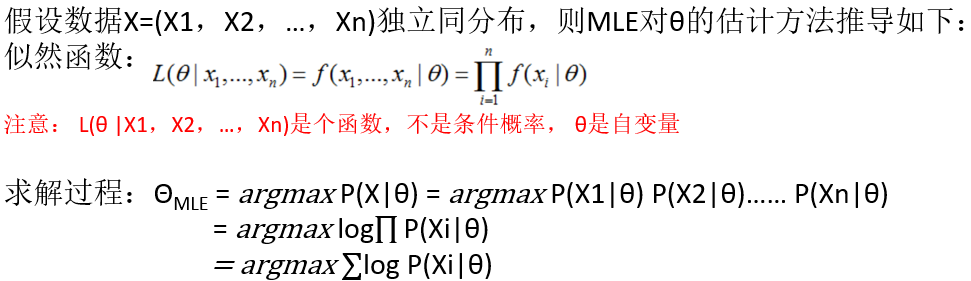求解过程

写出似然函数L(θ|x1,x2,x3,…)；
如果无法直接求导的话，对似然函数取对数，再平均（平均对数似然函数中有 1/n 项，消除了样本数量的影响），求解平均对数似然函数；
求导数 ；
求解模型中参数的最优值。

最大后验估计
概述
最大后验概率估计MAP：Maximum a posteriori estimation。
最大后验概率估计也是一种给定观察数据（X）来评估模型参数（θ）的方法，通过调整模型参数使得模型能够产生该数据样本的概率最大。与MLE不同的是，MAP对于模型参数有了一个先验假设，即模型参数可能满足某种分布，不再一味地依赖数据样例。
MAP是贝叶斯学派常用的估计方法，贝叶斯学派认为参数是随机值，因为没有观察到，那么和是一个随机数也没有什么区别，因此参数也可以有分布。
前提假设
假如x1,x2,x3,…每次独立抽样的概率模型中的参数θ不是一个固定值，而是一个符合g(θ)概率分布（先验概率）的随机变量。
核心思想
以当前样本数据条件下由贝叶斯公式计算出的整个后验概率P(θ|x1, x2, x3, …) 最大的模型参数θ为最终的模型参数。
（注意对比：最大似然估计是以让当前样本的概率最大的模型参数θ为最终的模型参数。）
推导过程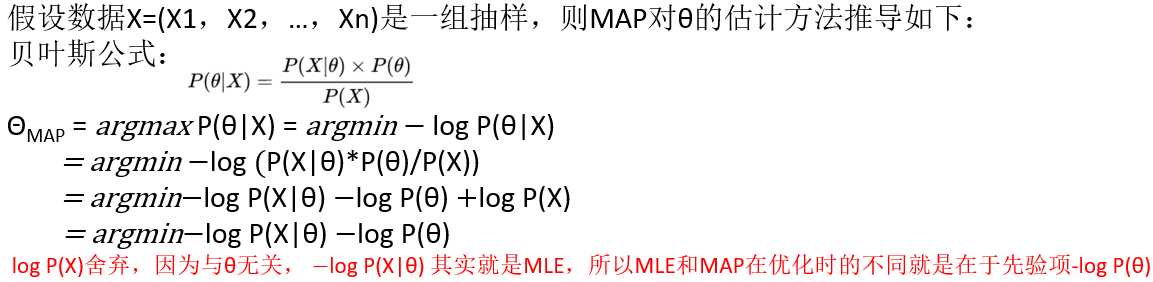贝叶斯公式：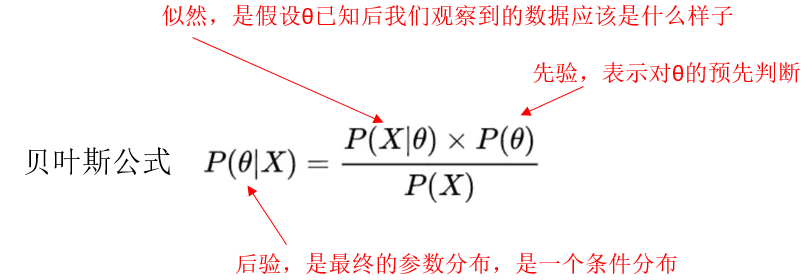贝叶斯估计
MAP作为贝叶斯估计的一种近似解，在贝叶斯估计中如果我们采用极大似然估计的思想，考虑后验分布极大化而求解，就变成了最大后验估计。
对比如下：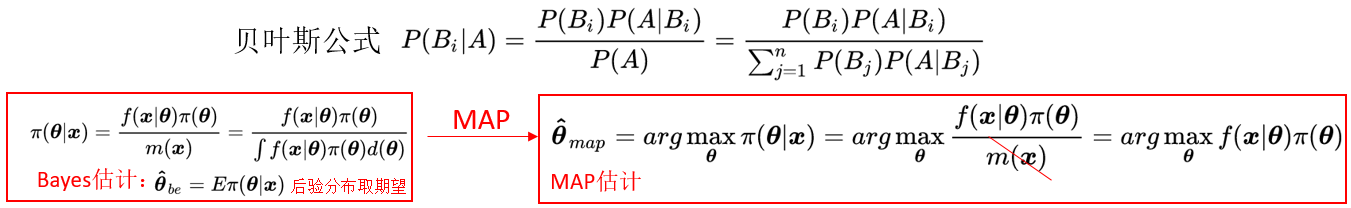最大似然估计和最大后验估计的对比
1.从上述推导过程可以看出，后验概率其实是在似然函数的基础上还考虑了先验概率的影响。两者的最大区别是MAP中加入了模型参数本身的概率分布g(θ)。
2.MLE中认为模型参数本身的概率的是均匀的，即该概率为一个固定值。当MAP中模型参数θ的先验概率为常数（固定值）时，问题就回到了MLE。
3.最大似然估计体现是的频率学派的观点，而最大后验估计体现的是贝叶斯学派的观点。
4.样本少的时候，MAP加入先验知识会更有用，随着样本数据量的增加，参数分布会越来越向数据靠拢，先验P(θ)的影响力会越来越小，MAP趋向等价于MLE，如果先验P(θ)=常数，其实本质上表示对事物没有任何预判。


展开全文• 分别解释了先验概率，后验概率，似然函数，还讲了最大似然估计和最大后验估计。 2.https://blog.csdn.net/qq_40213457/article/details/82502105 最大似然和最大后验讲的挺好的，MAP就是在MLE上加一个先验概率，或...
1.https://blog.csdn.net/qq_23947237/article/details/78265026
分别解释了先验概率，后验概率，似然函数，还讲了最大似然估计和最大后验估计。
2.https://blog.csdn.net/qq_40213457/article/details/82502105
最大似然和最大后验讲的挺好的，MAP就是在MLE上加一个先验概率，或MLE就是将先验概率视为1(视$\theta$为均匀分布)。

转载于:https://www.cnblogs.com/w-j-c/p/10834863.html
展开全文• 最大似然函数（MLE）和最大后验概率估计（MAP）是两种完全不同的估计方法，最大似然函数属于频率派统计（认为存在唯一真值 θ），最大后验估计属于贝叶斯统计（认为 θ 是一个随机变量，符合一定的概率分布），这是...
前言
本系列文章为 《Deep Learning》 读书笔记，可以参看原书一起阅读，效果更佳。
MLE VS MAP
最大似然函数（MLE）和最大后验概率估计（MAP）是两种完全不同的估计方法，最大似然函数属于频率派统计（认为存在唯一真值 θ），最大后验估计属于贝叶斯统计（认为 θ 是一个随机变量，符合一定的概率分布），这是两种认识方法的差异。模型不变，概率是参数推数据，统计是数据推参数。
最大似然估计
似然函数是一种关于模型中参数的函数，是根据模型的观测值，估计模型中参数的值。给定输出 x ，关于 θ 的似然函数 L(θ|x) 数值上等于给定参数 θ 后变量 X 的概率。其数学定义为：
$L(θ|x)=f_θ(x)=P_θ(X=x)$
最大似然估计是其中的一种好的估计，在样本趋近于无穷时，最大似然是收敛率最好的渐进估计，且由于它的一致性和统计效率，在机器学习中也是首选的估计方法。在独立同分布情况下：
$\hatθ_{MLE}=argmaxP(X;θ)=argmaxP(x_1;θ)P(x_2;θ)...P(x_n;θ) =argmax\log\prod_{i=1}^nP(x_i;θ)\\\\=argmax\sum_{i=1}^n\log P(x_i;θ) =argmin-\sum_{i=1}^n\log P(x_i;θ)//负对数似然$
由于对数函数单调增，因此想要求 L 的最大值，可以求其对数作为求其最大值的函数，这样求出的结果是相同的。深度学习所做分类任务中用到的交叉熵本质是求最大似然函数。
条件最大似然估计
$\hatθ_{MLE}=argmaxP(Y|X;θ)=argmax\sum_{i=1}^{m}\log{P(y^{(i)}|x^{(i)}|θ)}$
最大后验估计
贝叶斯公式：
$P(θ|x)=\frac{P(x|θ)P(θ)}{P(x)}$
其中 P(x|θ) 是似然函数，P(θ) 是先验概率。
则最大后验估计的数学定义为：
$\hat \theta_{MAP}(x)=\arg \max_\theta f(\theta|x)=\arg \max_\theta \frac{f(x|\theta)g(\theta)}{\int_\vartheta f(x|\vartheta)g(\vartheta)d\vartheta}=\arg\max_\theta f(x|\theta)g(\theta)$
theta 为需要估计的参数，f 为概率，g 为先验估计，最大化后验估计通过 f·g 求得。当先验分布为常数时，最大后验估计与最大似然估计重合。
总结
最大似然估计与最大后验估计对比分析。


展开全文机器学习 人工智能 数据分析 深度学习 python
• 两篇博文，图文并茂 https://blog.csdn.net/zengxiantao1994/article/details/72787849... ...   Wikipedia 关于最大后验估计和最大似然估计，基于贝叶斯推理，最大似然估计是最大后验估计在假设模型参数分布为均匀...
• 摘要最大似然估计(Maximum Likelihood Estimation)与最大后验估计(Maximum A Posteriori)是机器学习中最常用的两种点估计参数估计方法. 最大似然估计以最大化观测数据集上的似然度为目标, 强调从观测数据集上拟合出...
• （1）最大似然估计ML和最大后验估计MAP最大似然估计量非贝叶斯方法通常是最大化似然函数： 其中 被称为 的最大似然估计量，它是 的函数。最大后验估计量估计随机参数的通常方法是最大化后验分布函数： 其中 被称为 ...
• 它与Fisher的最大似然估计（Maximum Likelihood，ML）方法相近，不同的是它扩充了优化的目标函数，其中融合了预估计量的先验分布信息，所以最大后验估计可以看作是正则化（regularized）的最大似然估计。 理解： ...
• 最大后验估计 记录n次随机试验 Ω={ω1,⋯,ωn}出现正面的次数为m假设正面出现的概率为 θ=p(正面) 那么产生随机试验结果的概率为: p(Ω|θ) 式中 θ为自变量，频率学派认为这个概率θ最优解应该使得取最大值。在...
• 常用的参数估计方法包括最大似然估计法、最大后验估计、期望最大化法 (EM) 和贝叶斯估计方法。2 先验概率在观测数据前，我们将 θ 的已知知识表示成先验概率分布，p(θ) 我们通常称为先验。一般而言，在机器学习实践...
• 最大似然估计与最大后验估计(贝叶斯估计)频率学派 - Frequentist - Maximum Likelihood Estimation (MLE，最大似然估计)贝叶斯学派 - Bayesian - Maximum A Posteriori (MAP，最大后验估计)高中老师告诉我们概率就是...
• 最大似然估计、最大后验估计、贝叶斯估计的关系与区别机器学习
• 一、区别与联系 ...最大后验估计（MAP）是贝叶斯学派观点，有后验概率。 二、疑问 至今贝叶斯统计大量应用都是基于无信息先验进行的。那么贝叶斯统计还有什么意义呢，不就退化成似然估计了么？ ...
• ## 最大后验估计(MAP)

万次阅读 2018-12-07 10:55:26
最大后验估计（maximum a posteriori probability estimate, 简称MAP） 与最大似然估计类似，但是，在似然函数后面多乘了一项，即“待估计参数的先验分布”。故最大后验估计可以看作规则化的最大似然估计。 根据...
• 机器学习算法思想中,最大似然估计，最大后验估计经常遇见，必须掌握。 一.频率派与贝叶斯学派 二.最大似然估计(MLE) 三.最大后验估计(MAP) 一.频率派与贝叶斯学派 对于概率看法不同有两大派别,分别是频率学派与...
• 最大似然估计、最大后验估计、贝叶斯估计的对比 - 微笑sun - 博客园​www.cnblogs.com贝叶斯估计、最大似然估计、最大后验概率估计​www.jianshu.com好文共享。1、贝叶斯公式　这三种方法都和贝叶斯公式有关，所以...
• 贝叶斯学派 - Bayesian - Maximum A Posteriori (MAP，最大后验估计) 两大学派的争论 抽象一点来讲，频率学派和贝叶斯学派对世界的认知有本质不同：频率学派认为世界是确定的，有一个本体，这个本体的真值是不变的...机器学习 最大似然函数
• 机器学习的核心思想是从过往的经验中学习出规则，从而对新的事物进行预测。对于监督学习来说，有用的样本数目越多，训练越准确。...最大似然，最大后验估计都是给定模型参数后，得到样本集的概率的方法。贝叶斯思想
• 贝叶斯估计和最大后验估计 发表于1年前(2015-01-03 11:48) 阅读（23） | 评论（0） 0人收藏此文章, 我要收藏 赞0 使用贝叶斯估计计算参数比较困难，所以简化为最大后验估计。  最大后验估计...
• 在推导逻辑回归的损失...但在现实世界中，往往我们对参数是存在一定的先验知识的，而最大似然估计法则完全抛弃了这些先验知识，仅依靠观测数据做参数估计，因此很容易对数据形成过拟合，即在观测数据上表现非常好，...
• 然后就可以通过这个公式来求解最大似然估计MLE、最大后验估计MAP和贝叶斯估计了。 最大似然估计：实际上是求了红线框起来的部分。认为参数是固定的 最大后验估计：，实际上是去求了红线框起来的部分。比最大似然...
• 最大似然估计（Maximum Likelihood Estimation，MLE） 和 最大后验估计（Maximun A Posterior，MAP） - 介绍 MLE 和 MAP 方法用于参数估计的大致步骤。 - 详细介绍 MLE 和 MAP，包括其原理和计算过程。 - 讨论 ...
• 在机器学习中，总是会提到最大似然估计MLE(Maximum Likelihood Estimation)与最大后验估计MAP(Maximum A Posteririo)，我总是不明白其中的差别，我们求解问题不就是使用贝叶斯公式求解最大后验估计吗？这个和最大...
• ML-最大似然估计 maximum likelihood estimation ...MAP-最大后验估计 maximum a posterior estimation 贝叶斯估计 bayesian estimation 三者的关系及区别 https://www.cnblogs.com/little-YTMM/p/5399532.html朴素贝叶斯算法 算法
• 2.最大后验估计MAP：核心公式——条件概率公式 P(thita|x) = (P(x|thita)*P(thita))/P(x) 这里求令P(thita|x)最大的thita,和P(x)无关则约去。所以MAP最大化的函数相比于MLE多了一个thita的先验分布P(thita), 如果P...
• 最大似然和最大后验一、频率派与贝叶斯学派二、最大似然估计(MLE)三、最大后验估计(MAP)四、他们之间的联系 在机器学习算法中，最大似然和最大后验实在损失函数中很重要的一部分。 一、频率派与贝叶斯学派 对于概率......